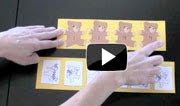## Sunday, November 28, 2010

### Preschool and Kindergarten Math

MATH WORKSHEETS
Free Math Worksheets
Our philosophy has been more focused on providing hands-on learning experiences but sometimes worksheets do come in handy. In the link below you will find free math worksheets that you can use in your classroom.

Free Math Worksheets

MATH CURRICULUM BEING DEVELOPED
Currently at kinderplans.com we are working on completing a full math program that can be used in conjunction with current themes or used on its own. It will cover all the math strands outlined below:
Matching, Sorting and Classifying
1. Identify, sort and classify objects by a specific attribute.
2. Patterning

Number Sense
1. Compare sets of objects if equal, more than, or less than (up to 10).
2. Print numbers 1-10
3. Count, recognize, represent, name, and order a number of objects (up to 30)
4. Use concrete objects to describe simple addition and subtraction to 10.

Measuring and Comparing
Geometry(Shapes)
1. Compare the length, weight and capacity of objects (e.g., longer, shorter, taller, lighter, heavier or holds more).
2. Demonstrate and understanding of time (e.g., morning, afternoon, evening, today, yesterday, tomorrow, week, year).
3. Identify time to the nearest hour.
4. Identify and describe common geometric objects (e.g. circle, triangle, square, rectangle, cube, sphere and cone).

Statistics, Data Analysis and Probability
Activities for graphing, collecting data and probability will be spread throughout the lessons.

## Friday, November 12, 2010

### Preschool and Kindergarten Math ActivitiesClick on Video to View Activities to Meet the Curriculum Outlines

MATH OUTCOMES

Outlined below are math outcomes for Kindergarten, however, this does differ according to the country or state. It does cover a broad spectrum so this can be adjusted to your situation. Also below is a link to free activities that cover these various strands. The video outlines these activities.
Matching, Sorting and Classifying
1. Identify, sort and classify objects by a specific attribute.
2. Patterning
Number Sense
1. Compare sets of objects if equal, more than, or less than
(up to 10).

2. Print numbers 1-10
3. Count, recognize, represent, name, and order a number
of objects (up to 30)

4. Use concrete objects describe simple addition and
subtraction to 10.
Measuring and Comparing
Geometry(Shapes)
1. Compare the length, weight and capacity of objects
(e.g., longer, shorter, taller, lighter, heavier or holds more).

2. Demonstrate and understanding of time
(e.g., morning, afternoon, evening, today, yesterday,
tomorrow, week, year).

3. Identify time to the nearest hour.
4. Identify and describe common geometric objects
(e.g. circle, triangle, square, rectangle, cube, sphere and cone).

Statistics, Data Analysis and Probability
Activities for graphing, collecting data and probability will be